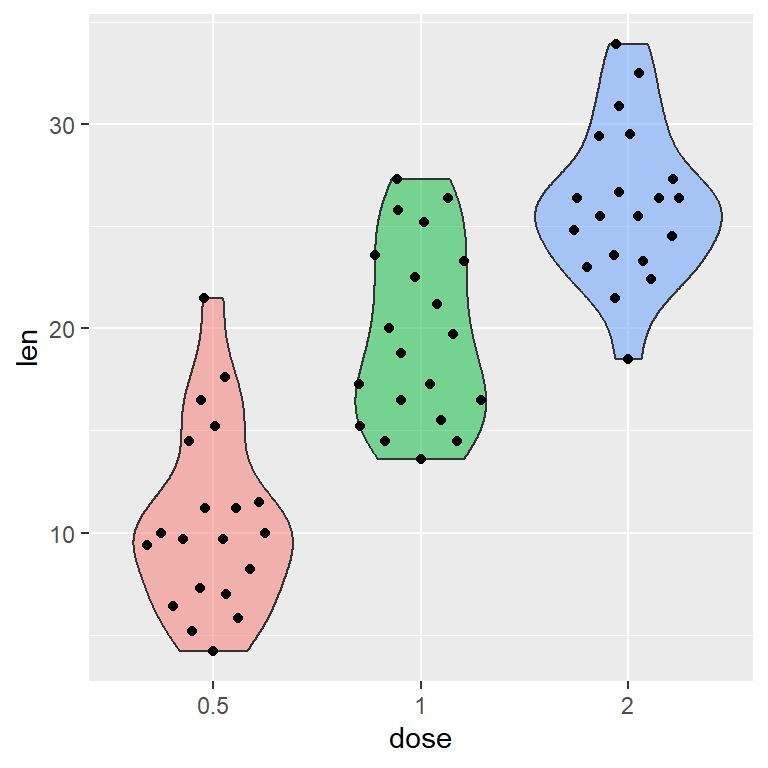# Violin plot with data points in ggplot2

## Sample data

The following data set contains the effect of vitamin C on Tooth Growth. The `dose` column has been converted into a factor to be used as the categorical variable.

``````# Sample data set
df <- ToothGrowth
df\$dose <- as.factor(df\$dose)``````

Option 1

The points can be added over a violin plot with `geom_point`. However, it is recommended to add some jitter with `position_jitter`, where `seed` is the pseurodandom number generator seed (optional) and `width` is the jittering width.

``````# install.packages("ggplot2")
library(ggplot2)

ggplot(df, aes(x = dose, y = len, fill = dose)) +
geom_violin(alpha = 0.5) +
geom_point(position = position_jitter(seed = 1, width = 0.2)) +
theme(legend.position = "none")``````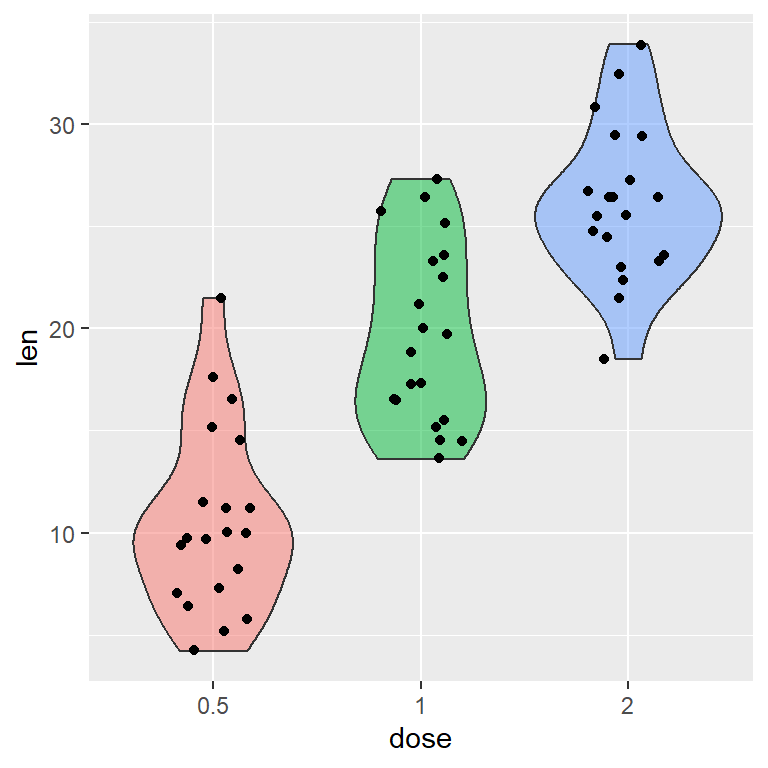Option 2

You can get the same result using `geom_jitter` function.

``````# install.packages("ggplot2")
library(ggplot2)

ggplot(df, aes(x = dose, y = len, fill = dose)) +
geom_violin(alpha = 0.5) +
geom_jitter(position = position_jitter(seed = 1, width = 0.2)) +
theme(legend.position = "none")``````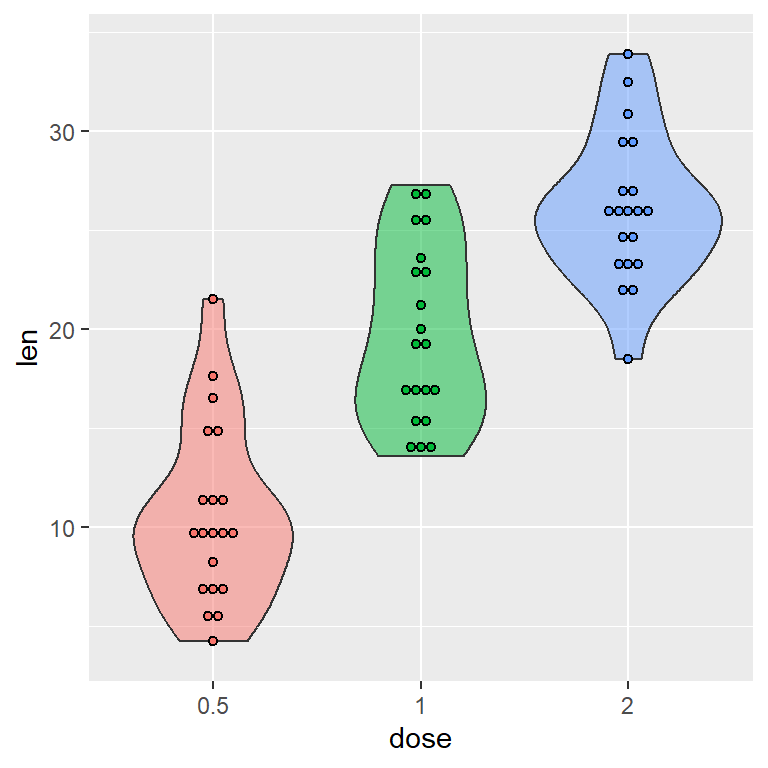If you want to create a violin plot with dots in ggplot2 you can use `geom_dotplot`, setting `binaxis = "y"` and `stackdir = "center"`. Note that `dotsize` controls the size of the points.

``````# install.packages("ggplot2")
library(ggplot2)

ggplot(df, aes(x = dose, y = len, fill = dose)) +
geom_violin(alpha = 0.5) +
geom_dotplot(binaxis = "y",
stackdir = "center",
dotsize = 0.5) +
theme(legend.position = "none")``````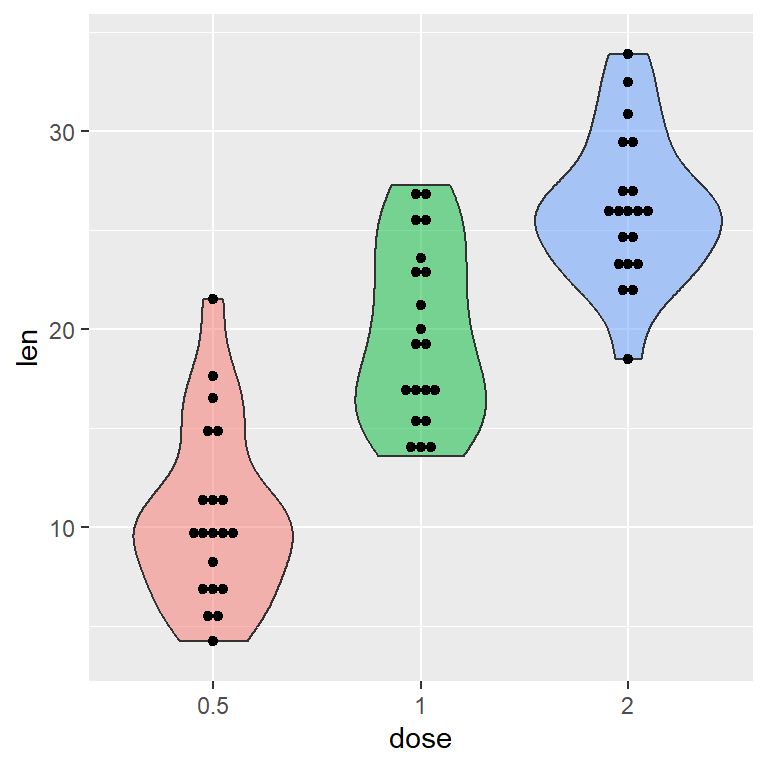The default dots are of the same color of the groups. To override this you can specify a fill color inside `geom_dotplot`.

``````# install.packages("ggplot2")
library(ggplot2)

ggplot(df, aes(x = dose, y = len, fill = dose)) +
geom_violin(alpha = 0.5) +
geom_dotplot(binaxis= "y",
stackdir = "center",
dotsize = 0.5,
fill = 1) +
theme(legend.position = "none")``````

An alternative for adding data points over a violin plot in ggplot is using the `geom_beeswarm` function from `ggbeeswarm` library.

``````# install.packages("ggplot2")
# install.packages("ggbeeswarm")
library(ggplot2)
library(ggbeeswarm)

ggplot(df, aes(x = dose, y = len, fill = dose)) +
geom_violin(alpha = 0.5) +
geom_beeswarm() +
theme(legend.position = "none")``````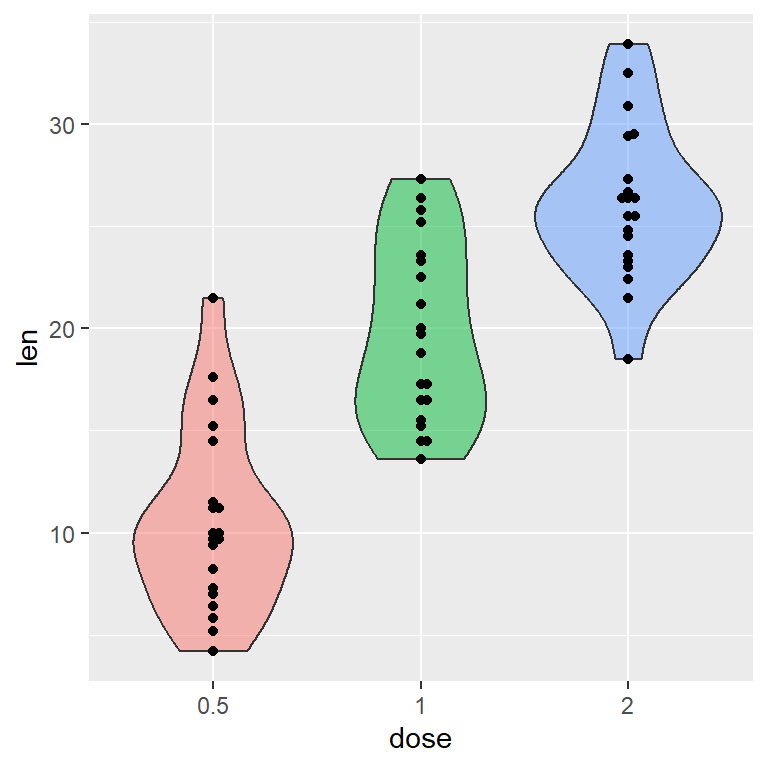The `ggbeeswarm` package also contains a function named `geom_quasirandom`, which can be used to add the points inside the violins, as you can see in the example below.

``````# install.packages("ggplot2")
# install.packages("ggbeeswarm")
library(ggplot2)
library(ggbeeswarm)

ggplot(df, aes(x = dose, y = len, fill = dose)) +
geom_violin(alpha = 0.5) +
geom_quasirandom() +
theme(legend.position = "none")``````